#### Chapter 8 Lines and Angle R.D. Sharma Solutions for Class 9th Math Exercise 8.4

Exercise 8.4

1. In Fig. 8.105, AB CD and ∠1 and ∠2 are in the ratio 3:2 Determine all angles form 1 to 8.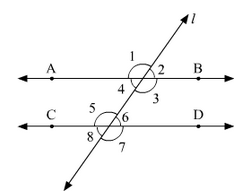Solution

Let ∠1 = 3x and ∠2 = 2x
∠1and∠2 are linear pair of angle
Now, ∠1and∠2
3x + 2x = 180
5x = 180
x = 180 / 5
x = 36 ∠1 = 3x = 108°,∠2 = 2x = 72°
We know, Vertically opposite angles are equal ∠1=∠3=108°,
∠2=∠4=72°,
∠6=∠7=108°,
∠5=∠8=72°
We also know, corresponding angles are equal ∠1=∠5=108°,
∠2=∠6=72°

2. In Fig. 8.106, l, m and n are parallel lines intersected by transversal p at X, Y and Z respectively. Find ∠1, ∠2 and ∠3.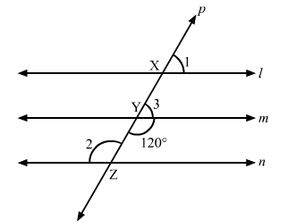Solution

From the given figure :
∠3+∠m YZ=180° [Linear pair]
∠3=180° –120°
∠3=60°
Now line l parallel to m
∠1=∠3 [Corresponding angles] ∠1=60°
Also m parallel to n,
∠2=120° [Alternative interior angle]
Hence, ∠1=∠3=60°

3. In Fig. 8.107, AB || CD || EF and GH || KL. Find the ∠HKL.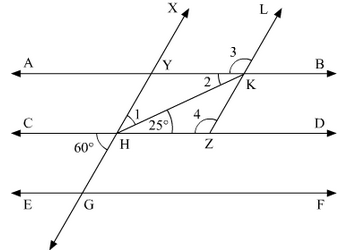Solution

Produce LK to meet GF at N.
Now, alternative angles are equal
∠CHG =∠HGN = 60
°
∠HGN =∠KNF = 60°    [Corresponding angles]
Hence, ∠KNG =180 - 60 = 120

∠GNK =∠AKL =120°  [Corresponding angles]
∠AKH =∠KHD =25°         [alternative angles]
Therefore, ∠HKL =∠AKH+∠AKL = 25+120 =145
°

4. In Fig. 8.108, show that AB || EF.Solution

Produce EF to intersect AC at K.
Now, ∠DCE+∠CEF=35+145=180°
Therefore, EF || CD (Since Sum of Co-interior angles is 180) —–(1)
Now, ∠BAC=∠ACD=57°
BA || EF [Alternative angles are equal] —–(2)
From (1) and (2)
AB || EF [Since, Lines parallel to the same line are parallel to each other]
Hence proved.

5. In Fig. 8.109, if AB || CD and CD || EF, find ∠ACE.Solution

Since EF || CD
Therefore, ∠EFC + ∠ECD = 180° [co-interior angles are supplementary]
⇒ ∠ECD = 180 - 130 = 50°
Also BA || CD
⇒ ∠BAC = ∠ACD = 70 [alternative angles]
But, ∠ACE + ∠ECD = 70°
⇒ ∠ACE = 70 - 50 = 20°

6. In Fig. 8.110, PQ || AB and PR || BC. If ∠QPR = 102°, determine ∠ABC. Give reasons.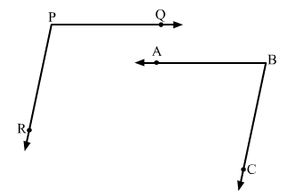Solution

AB is produce to meet PR at K
Since PQ || AB
∠QPR=∠BKR=102°    [corresponding angles]
Since PR || BC
∠RKB+∠CBK=180°   [ Since Corresponding angles are supplementary]
⇒ ∠CKB=180 -102=78
∴ ∠CKB=78°

7. In Fig. 8.111, state which lines are parallel and why.Solution

Vertically opposite angles are equal.
⇒ ∠EOC = ∠DOK=100°
∠DOK = ∠ACO = 100°
Here, two lines EK and CA cut by a third line and the corresponding angles to it are equal Therefore, EK || AC.

8. In Fig. 8.112, if l || m, n || p and ∠1 = 85°, find ∠2.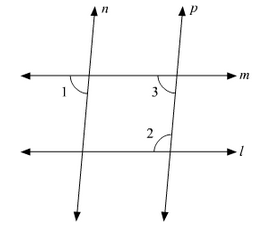Solution

Corresponding angles are equal
⇒ ∠1=∠3=85°
By using the property of co-interior angles are supplementary
∠2+∠3=180°
⇒ ∠2+55=180
⇒ ∠2=180-85
⇒ ∠2=95°

9. If two straight lines are perpendicular to the same line, prove that they are parallel to each other.

SolutionGiven m perpendicular t and l perpendicular to t.
∠1=∠2=90°
Since,  I and m are two lines and it is transversal and the corresponding angles are equal
L || M
Hence, proved.

10. Prove that if the two arms of an angle are perpendicular to the two arms of another angle, then the angles are either equal or supplementary.

Solution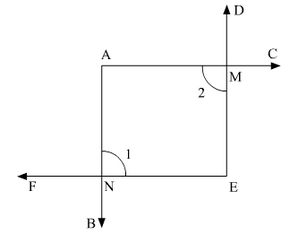Consider angles AOB and ACB.
Given,
OA perpendicular to AO,  also OB perpendicular to BO.
To prove: ∠AOB+∠ACB=180° (or) ∠AOB+∠ACB=180°
Proof :
[Sum of angles of quadrilateral is 360]
⇒ 180+ O +B + C = 360°
⇒ O+ C = 360 –180
Hence AOB + ACB = 180°     —–(1)
Also, B + ACB = 180
⇒ ACB =180 – 90 = ACES = 90°    —–(2)
From (i) and (ii), ACB = A0B = 90
Hence, the angles are equal as well as supplementary.

11. In Fig. 8.113, lines AB and CD are parallel and P is any point as shown in the figure. Show that ∠ABP + ∠ CDP = ∠DPB.Solution

Given that, AB ||CD
Let EF be the parallel line to AB and CD which passes through P.
It can be seen from the figure.
Alternative angles are equal.
∠ABP=∠BPF
Alternative angles are equal
∠CDP=∠DPF
ABP+∠CDP=∠BPF+∠DPF
∠ABP+∠CDP=∠DPB
Hence, proved.

AB parallel to CD, P is any point
To prove: ∠ABP+∠BPD+∠CDP=360°
Construction : Draw EF || AB passing through P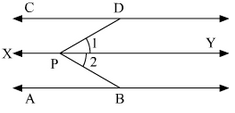Proof : Since, AB || EF and AB || CD.
Therefore, EF || CD
[Lines parallel to the same line are parallel to each other)
∠ABP+∠EPB=180°  [Sum of co-interior angles is 180)
⇒ ∠EPD+∠COP=180° - (1)  (Sum of co-interior angles is 180°)
⇒ ∠EPD+∠CDP=180° - (2)
∠ABP+∠EPB+∠EPD+∠CDP = (180+180)°
⇒ ∠ABP+∠EPB+∠COP = 360°

12. In Fig. 8.114, AB || CD and P is any point shown in the figure. Prove that:
∠ABP + ∠BPD + ∠CDP = 360°Solution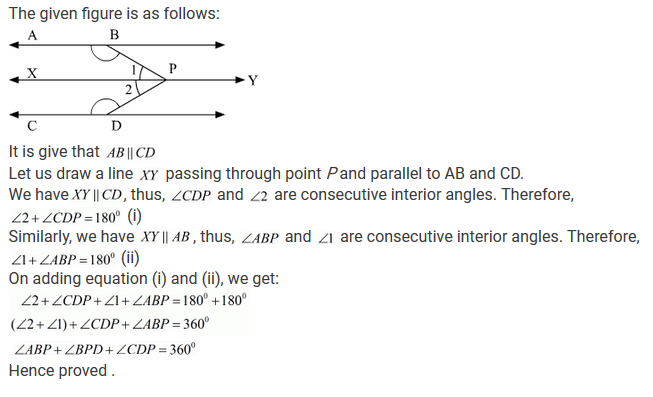13. Two unequal angles of a parallelogram are in the ratio 2 : 3. Find all its angles in degrees.Solution

Let A = 2x and B = 3x
Now, A+B = 180  [Co-interior angles are supplementary]
2x  + 3x = 180   [AD II BC and AB is the transversal)
⇒ 5x = 180
⇒ x = 180/5
⇒ x = 36
Therefore, A = 2×6 = 72
b = 3×36 = 108
Now, A = C = 72      [Opposite side angles of a parallelogram are equal)
B = D = 108

14. In each of the two lines is perpendicular to the same line, what kind of lines are they to each other?

Solution

The figure can be drawn as follows: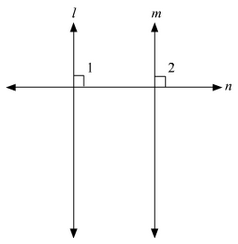Here, l⟂n and m⟂n.
We need to find the relation between lines l and m
It is given that l⟂n, therefore,
∠1 = 90° --- (i)
Similarly, we have m⟂n, therefore,
∠2 = 90° --- (i) (ii)
From (i) and (ii), we get:
∠1 = 2
But these are the pair of corresponding angles.
Theorem states: If a transversal intersects two lines in such a way that a pair of corresponding angles is equal, then the two lines are parallel.
Thus, we can say that l∥m.
Hence, the lines are parallel to each other.

15. In Fig. 8.115, ∠1 = 60° and ∠2 = (2/3) rd of a right angle. Prove that l∥m.Solution

The figure is given as follow: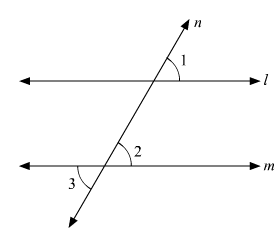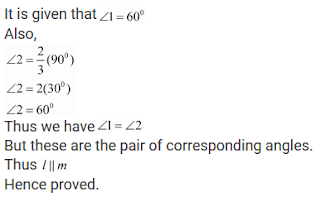16. In Fig. 8.116, if l || m || n and ∠1 = 60°, find ∠2.Solution

Since l parallel to m and p is the transversal
Therefore, Given: l||m||n ∠l = 60°
To find ∠2
∠1=∠3=60° [Corresponding angles]
Now, ∠3and∠4 are linear pair of angles ∠3+∠4=180°
60 + ∠4 = 180
∠4 = 180 - 60
⇒ 120
Also, m||n and P is the transversal
Therefore ∠4 = ∠2 =120 [Alternative interior angle]
Hence 2 ∠2 = 120

17. Prove that the straight lines perpendicular to the same straight line are parallel to one another.

SolutionHere, l⟂n and m⟂n.
We need to prove that l∥m
It is given that l⟂n, therefore,
∠1 = 90° ---(i)
Similarly, we have m⟂n, therefore,
∠2 = 90° --- (ii)
From (i) and (ii), we get:
∠1 = ∠2
But these are the pair of corresponding angles.
Theorem states: If a transversal intersects two lines in such a way that a pair of corresponding angles is equal, then the two lines are parallel.
Thus, we can say that l∥m.

18. The opposite sides of a quadrilateral are parallel. If one angle of the quadrilateral is 60°, find the other angles.

Solution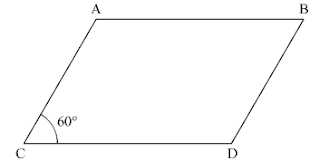Given AB || CD
Since AB || CD and AD is the transversal
Therefore, A + D = 180 (Co-interior angles are supplementary)
60 + D = 180
D = 180 - 60
D = 120
Now. AD || BC and AB is the transversal
A + B = 180 (Co-interior angles are supplementary)
60 +B = 180
B = 180 - 60
= 120 Hence, ∠A=∠C=60° and∠B=∠D=120°

19. Two lines AB and CD intersect at O. If ∠AOC + ∠COB + ∠BOD = 270°, find the measures of ∠AOC, ∠COB, ∠BOD and ∠DOA.

SolutionGiven : ∠AOC+∠COB+∠BOD=270°
To find : ∠AOC,∠COB,∠BOD,∠DOA
Here, ∠AOC+∠COB+∠BOD=270° [ Complete angle]
270 + AOD = 360
AOD = 360 - 270
AOD = 90
Now, AOD + BOD = 180 [Linear pair]
90 + BOD = 180
BOD = 180 – 90
BOD = 90
AOD = BOC = 90 [Vertically opposite angles]
BOD = AOC = 90 [Vertically opposite angles]

20. In Fig. 8.117, p is a transversal to lines m and n, ∠2 = 120° and ∠5 = 60°. Prove that m || n.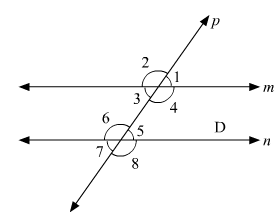Solution

Given that
∠2=120° and ∠5=60°
To prove,
∠2+∠1=180° [ Linear pair ] 120+∠1=180 ∠1=180−120 ∠1=60°
Since ∠1=∠5=60°
Therefore, m||n [As pair of corresponding angles are equal]

21. In Fig. 8.118, transversal l intersects two lines m and n, ∠4 = 110° and ∠7 = 65°. Is m || n ?Solution

Given :
∠4=110° and ∠7=65°
To find : Is m||n
Here. ∠7=∠5=65° [Vertically opposite angle]
Now. ∠4+∠5=110+65=175°
Therefore, m is not parallel to n as the pair of co interior angles is not supplementary.

22.  Which pair of lines in Fig. 8.119 are parallel ? Given reasons.Solution

∠A+∠B=115+65=180°
Therefore, AB || BC [As sum of co interior angles are supplementary]
∠B+∠C=65+115=180°
Therefore, AB || CD [As sum of interior angles are supplementary]

23. If I, m, n are three lines such that I|| m and n perpendicular to l, prove that n perpendicular to m.

Solution

The figure can be drawn as follows:Given,
l || m,
n perpendicular to l
To prove: n perpendicular to m
Since l || m and n intersects
∴ ∠1=∠2 [Corresponding angles]
But, ∠1 = 90
∠2=90°
Hence n is perpendicular to m.

24. In the below fig, arms BA and BC of ∠ABC are respectively parallel to arms ED and EF of∠DEF. Prove that ∠ABC=∠DEF.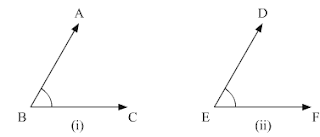Solution

Given,
AB || DE and BC || EF
To prove : ∠ABC=∠DEF
Construction:- Produce BC to x such that it intersects DE at M.
Proof :
Since AB || DE and BX is the transversal.
ABC = DMX [Corresponding angle] - (i)
Also, BX || EF and DE is the transversal
DMX = DEF [Corresponding angles] - (ii)
From (i) and (ii) ∠ABC=∠DEF

25. In Fig. 8.121, arms BA and BC of ∠ABC are respectively parallel to arms ED and EF of ∠DEF. Prove that ∠ABC + ∠DEF = 180°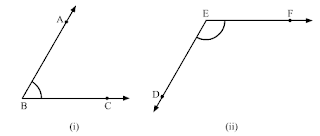Solution

Given:
AB∥DE,
BC∥EF
To prove: ∠ABC+∠DEF=180°
Construction: Produce BC to intersect DE at MProof :
Since AB || EM and BL is the transversal
∠ABC=∠EML [Corresponding angle] - (i)
Also,
EF || ML and EM is the transversal
By the property of co-interior angles are supplementary
∠DEF+∠EML=180° ---(ii)
From (i) and (ii),
Therefore, ∠DEF+∠ABC=180°

26. With of the following statements are true (T) and which are false (F)? Give reasons.
(i) If two lines are intersected by a transversal, then corresponding angles are equal.
(ii) If two parallel lines are intersected by a transversal, then alternate interior angles are equal.
(ii) Two lines perpendicular to the same line are perpendicular to each other.
(iv) Two lines parallel to the same line are parallel to each other.
(v) If two parallel lines are intersected by a transversal, then the interior angles on the same side of the transversal are equal.

Solution

(i) False
(ii)True
(iii) False
(iv) True
(v) False

28. Fill in the blanks in each of the following to make the statement true:
(i) If two parallel lines are intersected by a transversal, then each pair of corresponding angles are ...
(ii) If two parallel lines are intersected by a transversal, then interior angles on the same side of the transversal are ....
(iii) Two lines perpendicular to the same line are ... to each other.
(iv) Two lines parallel to the same line are ... to each other.
(v) If a transversal intersects a pair of lines in such away that a pair of alternate angles are equal, then the lines are ...
(vi) If a transversal intersects a pair of lines in such away that the sum of interior angles on the same side of transversal is 180°, then the lines are ...

Solution

(i) If two parallel lines are intersected by a transversal, then corresponding angles are equal.

(ii) If two parallel lines are intersected by a transversal, then interior angles on the same side of the transversal are supplementary.

(iii) Two lines perpendicular to the same line are parallel to each other.

(iv) Two lines parallel to the same line are parallel to each other.

(v) If a transversal intersects a pair of lines in such a way that a pair of interior angles is equal, then the lines are parallel.

(vi) If a transversal intersects a pair of lines in such a way that a pair of interior angles on the same side of transversal is 180° , then the lines are parallel.